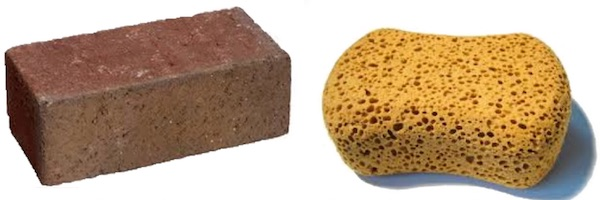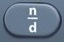# DensityBasics
Density refers to how tightly packed a particular object is.

In the above image, the brick has higher density than the sponge.

You should be given the following formula:
Density =$\bf\displaystyle\frac{Mass}{Volume}$

Question

A block of wood has a mass of 30 g and a volume of 10 cm³.
What is its density?

3 g/cm³

See Plug-In.
Mass = 30 g
Volume = 10 cm³

Density =$\bf\displaystyle\frac{Mass}{Volume}$

Density =$\bf\displaystyle\frac{30}{10}$ = 3

Input Display Commentblinker clears screen3010$\bf\displaystyle\frac{30}{10}$ Density =$\bf\displaystyle\frac{Mass}{Volume}$
Plug in 30 for Mass.
Plug in 10 for Volume.3 Answer
###### Density

Question

Five blocks of wood have the following properties:
Block 1:  Mass = 30 g; Volume = 10 cm³.
Block 2:  Mass = 28 g; Volume = 4 cm³.
Block 3:  Mass = 26 g; Volume = 13 cm³.
Block 4:  Mass = 24 g; Volume = 8 cm³.
Block 5:  Mass = 22 g; Volume = 11 cm³.

What is the mean (average) density of the five blocks of wood?

3.4 g/cm³

See Plug-In.
See Mean.

Density =$\bf\displaystyle\frac{Mass}{Volume}$

Mean =$\bf\displaystyle\frac{Sum}{Count}$

Input Display Commentblinker clears screen3010$\bf\displaystyle\frac{30}{10}$ Density =$\bf\displaystyle\frac{Mass}{Volume}$
Plug in 30 for Mass.
Plug in 10 for Volume.3 Density Block 1284$\bf\displaystyle\frac{28}{4}$ Density =$\bf\displaystyle\frac{Mass}{Volume}$
Plug in 28 for Mass.
Plug in 4 for Volume.7 Density Block 22613$\bf\displaystyle\frac{26}{13}$ Density =$\bf\displaystyle\frac{Mass}{Volume}$
Plug in 26 for Mass.
Plug in 13 for Volume.2 Density Block 3248$\bf\displaystyle\frac{24}{8}$ Density =$\bf\displaystyle\frac{Mass}{Volume}$
Plug in 24 for Mass.
Plug in 8 for Volume.3 Density Block 42211$\bf\displaystyle\frac{22}{11}$ Density =$\bf\displaystyle\frac{Mass}{Volume}$
Plug in 22 for Mass.
Plug in 11 for Volume.2 Density Block 53+7+2+3+25$\bf\displaystyle\frac{3+7+2+3+2}{5}$ Mean =$\bf\displaystyle\frac{Sum}{Count}$3.4 Answer
###### DensityMean

Practice – Questions

1.  A block of wood has a mass of 35 g and a volume of 10 cm³.  What is its density?
A.  1.5 g/cm³
B.  2.0 g/cm³
C.  2.5 g/cm³
D.  3.5 g/cm³

2.  A block of wood has a mass of 24 g and a volume of 3 cm³.  What is its density?
A.  4.5 g/cm³
B.  5.0 g/cm³
C.  8.0 g/cm³
D.  3.0 g/cm³

3.  A block of wood has a mass of 66 g and a volume of 6 cm³.  What is its density?
A.  11.0 g/cm³
B.  6.0 g/cm³
C.  10.0 g/cm³
D.  1.0 g/cm³

4.  A block of wood has a mass of 108 g and a volume of 12 cm³.  What is its density?
A.  12.0 g/cm³
B.  9.0 g/cm³
C.  8.0 g/cm³
D.  11.0 g/cm³

5.  To the nearest hundredth, what is the mean (average) density of the above four blocks of wood?
A.  31.50 g/cm³
B.  31.51 g/cm³
C.  7.87 g/cm³
D.  7.88 g/cm³